## Each side of a metal plate is illuminated by light of different wavelengths. The left side is illuminated by light with λ0 = 500 nm and the

Question

Each side of a metal plate is illuminated by light of different wavelengths. The left side is illuminated by light with λ0 = 500 nm and the right side by light of unknown λ. Two electrodes A and B provide the stopping potential for the ejected electrons. If the voltage across AB is VAB=1.2775 V, what is the unknown λ?

in progress 0
1 month 2021-08-12T13:22:36+00:00 1 Answers 0 views 0

The  wavelength is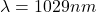Explanation:

From the question we are told that

The  wavelength of the left light is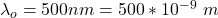The  voltage across A  and  B is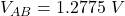Let the stopping potential  at A  be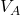and the electric potential at B  be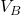The voltage across A and B is mathematically represented as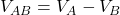Now  According to Einstein’s photoelectric equation the stopping potential at A for the ejected electron from the left side  in terms of electron volt is mathematically represented as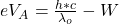Where  W is the work function of the metal

h is the Planck constant with values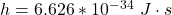c  is the speed of light with value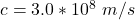And  the stopping potential at B for the ejected electron from the right side  in terms of electron volt is mathematically represented as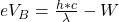So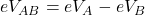=>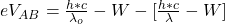=>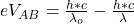=>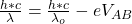=>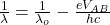Where e is the charge on an electron with the value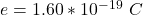=>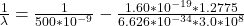=>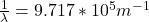=>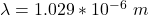=>